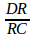Question Pool Parallel Lines

# Parallel Lines - SAMAGRA Question Pool & Answers | Class 9 English Medium

Kerala Syllabus SAMAGRA SCERT SAMAGRA Question Pool for Class 9 English Medium Maths Parallel LinesQn 1.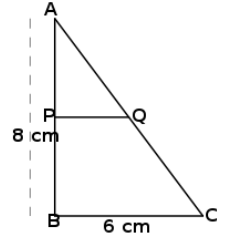In right triangle ABC, BC=6cm and AB=8cm. P is the mid point of AB and PQ is parallel to BC.

a) Find the length of AC

b) Write the length of three sides of triangle APQ

c) If AB=a, BC= b and AC=c, then Find the perimeters of ABC and APQ

Get Free Study Materials + 1 Week Free Trial of BrainsPrep Class 9 English Medium Tuition

Qn 2.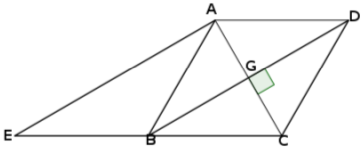In the figure ABCD is a rhombus . AE is parallel to BD. Prove that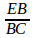=1

Get Free Study Materials + 1 Week Free Trial of BrainsPrep Class 9 English Medium Tuition

Qn 3.

Prove that the quadrilateral formed by joining the midpoints of the sides of a triangle is a rhombus.

Get Free Study Materials + 1 Week Free Trial of BrainsPrep Class 9 English Medium Tuition

Qn 4.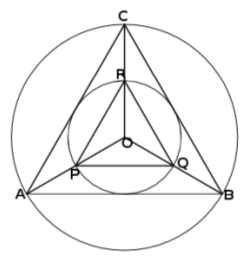O is the circum centre of the triangles ABC and PQR. OC=2xOR

Then – Radius of the larger circle is twice the radius of the small circle

a) AP : PO = ------

b) Are AC and PR parallel? Why?

c) If Δ ABC is an equilateral triangle? Prove that ΔPQR is also an equilateral triangle.

a)    AP: PO = 1 : 1

Get Free Study Materials + 1 Week Free Trial of BrainsPrep Class 9 English Medium Tuition

Qn 5.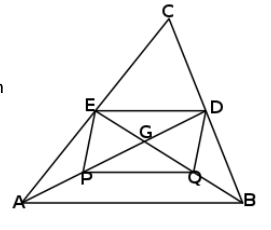In the picture below, the medians from the two bottom vertices intersect at G. AB=6cm.

a) Find the length of ED

b) Find the length of PQ joined by the mid points of AG and BG

c) Prove that EP = DQ

a) ED

Get Free Study Materials + 1 Week Free Trial of BrainsPrep Class 9 English Medium Tuition

Qn 6.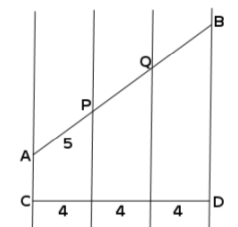In the figure four parallel lines cut lines AB and CD. If AP=5cm. Find the length of AB

AB= 5x3=15 cm

Get Free Study Materials + 1 Week Free Trial of BrainsPrep Class 9 English Medium Tuition

Qn 7.

Draw a rectangle with perimeter 24cm and sides in the ratio 3:2

Drawing  a line of length 12 cm

Drawing another line with 5 equal parts

Marking a point which

Get Free Study Materials + 1 Week Free Trial of BrainsPrep Class 9 English Medium Tuition

Qn 8.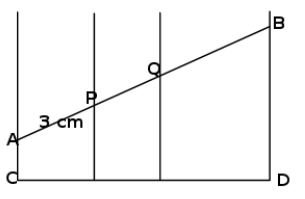In the figure four parallel lines cut CD in the ratio 2:3:4. If AP=3 cm find the length of AB.

Get Free Study Materials + 1 Week Free Trial of BrainsPrep Class 9 English Medium Tuition

Qn 9.

Draw an equilateral triangle of perimeter 8cm.

Drawing a line AC=8cm

For drawing another line from one end of the first line

Dividing the second line into 3 equal parts

Get Free Study Materials + 1 Week Free Trial of BrainsPrep Class 9 English Medium Tuition

Qn 10.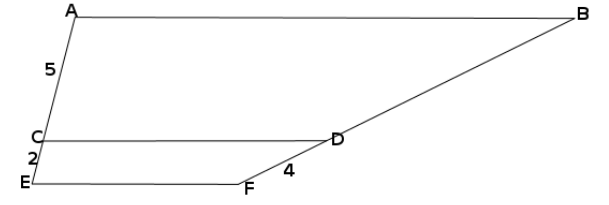AB, CD, EF are parallel lines. If AC= 5cm, CE=2cm, DF=4cm find the length of BD.

Get Free Study Materials + 1 Week Free Trial of BrainsPrep Class 9 English Medium Tuition

Qn 11.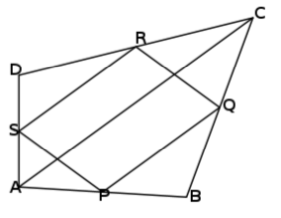Quadrilateral PQRS is drawn by joining the midpoints of the sides of the quadrilateral ABCD. AC= 10cm

a) Find the length of SR

b) Prove that PQRS is a parallelogram.

a) SR =

Get Free Study Materials + 1 Week Free Trial of BrainsPrep Class 9 English Medium Tuition

Qn 12.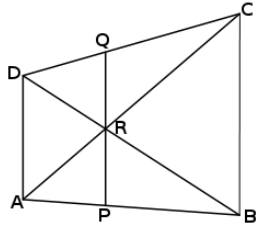In the figure AD, PQ and BC are parallel lines.

a) If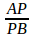=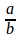, then what is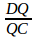and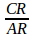b) Prove that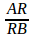=